## Magnetic Field

Magnetic Field

Magnets show repulsion or attraction force around itself. This area affected from the force of magnets called magnetic field. We cannot see magnetic field necked eye. However, if we put a sheet on the magnet and put some iron filing on this sheet we can easily observe the magnetic field around the magnet with the shapes of the iron filling. The shapes of magnetic field lines showed in the picture given below;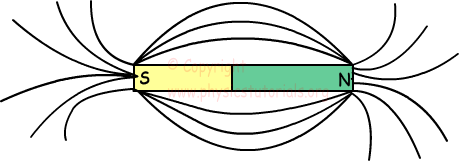Directions of magnetic field lines are showed below;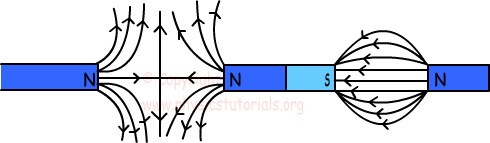When the lines get closer to each other, this means that magnetic field is strong in that region.

Magnetic field lines;

• Never intersect
• If they are parallel we say that there is a regular magnetic field.

Electricity and magnetism are closely related to each other. Electricity causes magnetic field. Scientists have observed that, when a compass is put next to the wire (wire connected to a battery and current flows), needle of the compass is deflected. The reason of this deflection is magnetic field and they conclude that electric current produces magnetic field. Magnetic field lines around a wire are shown below;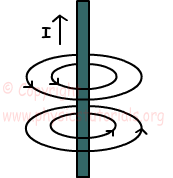Magnetic field is a vector quantity and showed with the letter B. Unit of B is Tesla. When we calculate magnetic field of a magnet we assume that there is a 1 unit of m at the point we want to find. We find the magnetic field with following formula;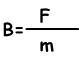Example: If k.m/d2 is equal to 5 N/Amp.m find the magnetic field produced by the m1 and m2 at point A.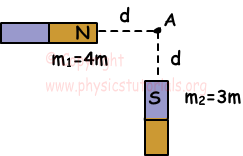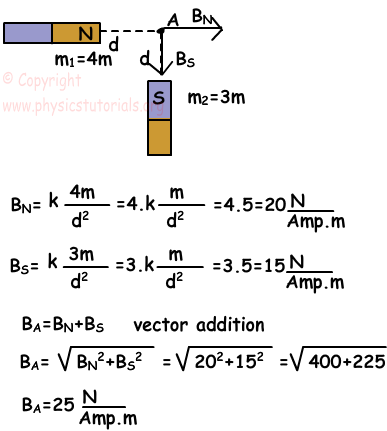Author: